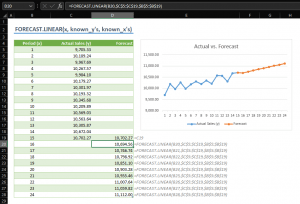The FORECAST.LINEAR function is a Statistical function that predicts a future value by using existing values along a linear trend (linear regression). You can use a forecasting function to predict future sales, inventory requirements, or consumer trends. In this guide, we’re going to show you how to use the FORECAST LINEAR function and go over some tips and error handling methods.

## FORECAST.LINEAR Function Syntax

FORECAST.LINEAR(x, known_y's, known_x's)

## Arguments

 x The data point to use to predict a value. known_y's The dependent array or range of data (y values). known_x's The independent array or range of data (x values).

## FORECAST.LINEAR Function Examples

The FORECAST.LINEAR function predicts the value of data point x by given historical known_y's values and known_x's data points.

In our example, our historical data resides in the range B5:C19. See that the cell D20 has the FORECAST.LINEAR to predict the value based on B20.

=FORECAST.LINEAR(B20,\$C\$5:\$C\$19,\$B\$5:\$B\$19)A single forecasted value will not tell you much. The best way to review and compare the forecasted data is to forecast for more points and use a line chart to see the trend. All you need to do is to generate more data points and copy the formula. Just pay attention to absolute and relative references, since you will want to keep ranges of the historical data.Note that the cell D19 has the same value as the last historical data just to connect lines in the graph.

## Excel Forecasting Functions

 Forecasting with Exponential Triple Smoothing FORECAST.LINEAR.ETS Seasonality in a forecasting using Exponential Triple Smoothing FORECAST.LINEAR.ETS.SEASONALITY Confidence interval in a forecasting using Exponential Triple Smoothing FORECAST.LINEAR.ETS.CONFINT Statistical values in a forecasting using Exponential Triple Smoothing FORECAST.LINEAR.ETS.STAT